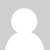## Interrupt based Pulse Counter

Example Arduino sketch for interrupt based pulse counting:

```//Number of pulses, used to measure energy.
long pulseCount = 0;   ```

//Used to measure power.
unsigned long pulseTime,lastTime;

//power and energy
double power, elapsedkWh;

//Number of pulses per wh - found or set on the meter.
int ppwh = 1; //1000 pulses/kwh = 1 pulse per wh

void setup()
{
Serial.begin(115200);

// KWH interrupt attached to IRQ 1 = pin3
attachInterrupt(1, onPulse, FALLING);
}

void loop()
{

}

// The interrupt routine
void onPulse()
{

//used to measure time between pulses.
lastTime = pulseTime;
pulseTime = micros();

//pulseCounter
pulseCount++;

//Calculate power
power = (3600000000.0 / (pulseTime - lastTime))/ppwh;

//Find kwh elapsed
elapsedkWh = (1.0*pulseCount/(ppwh*1000)); //multiply by 1000 to convert pulses per wh to kwh

//Print the values.
Serial.print(power,4);
Serial.print(" ");
Serial.println(elapsedkWh,3);
}

## Accuracy

Range of 0 to 25kW (typical range for solid state pulse output meters):

1000 pulses per kWh

 Pulse Gen (kW) Pulse Counter (kW) Error (W) % Error Std. Deviation 0.101 0.101 0.00 0.00% 0 2.56 2.5599 0.10 0.00% 0.00017 3.15 3.1496 0.40 0.00% 0.00049 6.89 6.888 2.00 0.00% 0.001 9.56 9.556 4.00 0.00% 0.0019 10.63 10.625 5.00 0.00% 0.0024 19.62 19.6 20.00 0.00% 0.008 22.56 22.535 25.00 0.00% 0.0111 24.98 24.95 30.00 0.00% 0.0126

## Notes

If the Serial monitor displays values rapidly, and at excessive power levels, try connecting a 10k pull down resistor to the digital input.### Re: Interrupt based Pulse Counter

I think the column headings above need adjusting, I was more than a bit confused when I read it the first time... :)